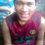Beat the Machines!

An advertisement for a symbolic algebra program claims that an engineer worked for three weeks on the integral $\int {(k \ln x-2x^3+3x^2+b)^4 \text{ d}x}$ which deals with turbulence in an aerospace application. The advertisement said that the engineer never got the same answer twice in three weeks.

1. Give two reduction formulas that will be of use in finding the above integral. Establish each formula.

2. Explain (only) how you could use those two reduction formulas in finding the integral, i.e. what is your strategy?

3. Challenge! With your strategy in #2, compute the engineer's integral by hand. You may comment only the final answer here, as the solution is very very lengthy.

4. Compare your answer with those produced by the following computer algebra systems. Use $k=b=1$. (Links to them are provided for easy access. More computer algebra systems will be added here later):

5. Is there any reason for the engineer to take three weeks?

[The problem information was taken from Edwards and Penney, $Calculus\text{ }6th\text{ }Edition$.]Note by Jaydee Lucero
5 years, 1 month ago

This discussion board is a place to discuss our Daily Challenges and the math and science related to those challenges. Explanations are more than just a solution — they should explain the steps and thinking strategies that you used to obtain the solution. Comments should further the discussion of math and science.

When posting on Brilliant:

• Use the emojis to react to an explanation, whether you're congratulating a job well done , or just really confused .
• Ask specific questions about the challenge or the steps in somebody's explanation. Well-posed questions can add a lot to the discussion, but posting "I don't understand!" doesn't help anyone.
• Try to contribute something new to the discussion, whether it is an extension, generalization or other idea related to the challenge.
• Stay on topic — we're all here to learn more about math and science, not to hear about your favorite get-rich-quick scheme or current world events.

MarkdownAppears as
*italics* or _italics_ italics
**bold** or __bold__ bold

- bulleted
- list

• bulleted
• list

1. numbered
2. list

1. numbered
2. list
Note: you must add a full line of space before and after lists for them to show up correctly
paragraph 1

paragraph 2

paragraph 1

paragraph 2

> This is a quote
This is a quote
# I indented these lines
# 4 spaces, and now they show
# up as a code block.

print "hello world"
# I indented these lines
# 4 spaces, and now they show
# up as a code block.

print "hello world"
MathAppears as
Remember to wrap math in $$...$$ or $...$ to ensure proper formatting.
2 \times 3 $2 \times 3$
2^{34} $2^{34}$
a_{i-1} $a_{i-1}$
\frac{2}{3} $\frac{2}{3}$
\sqrt{2} $\sqrt{2}$
\sum_{i=1}^3 $\sum_{i=1}^3$
\sin \theta $\sin \theta$
\boxed{123} $\boxed{123}$

Sort by:

$b^4 - 4 b^3 k + 12 b^2 k^2 - 24 b k^3 + 24 k^4) x + \frac{4}{9} (9 b^3 - 9 b^2 k + 6 b k^2 - 2 k^3) x^3 + \frac{1}{16} (-32 b^3 + 24 b^2 k - 12 b k^2 + 3 k^3) x^4 + \frac{54}{125} (25 b^2 - 10 b k + 2 k^2) x^5 - \frac{2}{3} (18 b^2 - 6 b k + k^2) x^6 + \frac{12}{343} (98 b^2 - 7 b (-63 + 4 k) + k (-63 + 4 k)) x^7 - \frac{27}{8} (8 b - k) x^8 + \frac{1}{9} (81 + 144 b - 16 k) x^9 - \frac {4}{25} (135 + 20 b - 2 k) x^10 + \frac{(216 x^11)}{11} - 8 x^12 +\frac{ ( 16 x^13)}{13} - (\frac{1}{14700}) k x (-58800 b^3 + 352800 k^3 + 1225 k^2 x^2 (-32 + 9 x) + 48 k x^4 (1323 - 1225 x + 300 x^2) + 88200 b^2 (2 k + (-2 + x) x^2) + 420 x^6 (-540 + 945 x - 560 x^2 + 112 x^3) - 420 b (840 k^2 + 35 k x^2 (-8 + 3 x) + 12 x^4 (63 - 70 x + 20 x^2))) Log[x] + \frac{1}{70} k^2 x (420 b^2 + 840 k^2 + 35 k x^2 (-8 + 3 x) + 12 x^4 (63 - 70 x + 20 x^2) - 420 b (2 k + (-2 + x) x^2)) Log[ x]^2 - 2 k^3 x (-2 b + 2 k + (-2 + x) x^2) Log[x]^3 + k^4 x Log[x]^4$

I think this is the answer as long as I didn't do any mistake in arithmetic calculations.

- 4 years, 6 months ago

That's interesting.

I'm working on it.

- 4 years, 6 months ago

Yup! It is indeed interesting . But I am more interested in guessing the train of thoughts in that engineer's mind .

It's always fascinating to find(deduce) what someone else thinks :D

- 4 years, 6 months ago